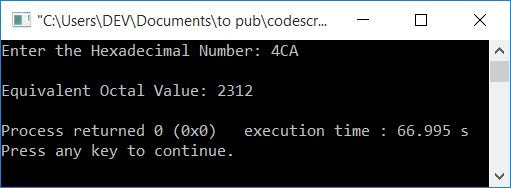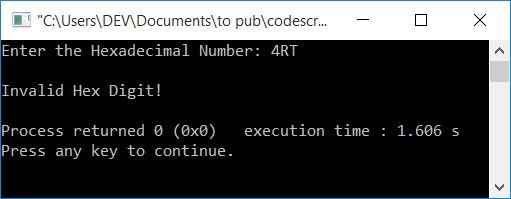# C++ Program to Convert Hexadecimal to Octal

In this article, you will learn about and get code for hexadecimal to octal conversion in C++. The program is written both with and without the use of functions.

Before going through the program, if you're not aware of the simple steps and formula used for the conversion, you can refer to the hexadecimal to octal steps and formula to get all the required information.

## Hexadecimal to Octal in C++

To convert a hexadecimal number to an octal number in C++ programming, you have to ask the user to enter the hexadecimal number first. And then convert it into its equivalent octal value. Print its equivalent octal value as shown in the program given below:

```#include<iostream>
#include<math.h>
using namespace std;
int main()
{
int decimalNum=0, octalNum, rem, i=0, len=0;
char hexDecNum;
cin>>hexDecNum;
while(hexDecNum[i]!='\0')
{
len++;
i++;
}
len--;
i=0;
while(len>=0)
{
rem = hexDecNum[len];
if(rem>=48 && rem<=57)
rem = rem-48;
else if(rem>=65 && rem<=70)
rem = rem-55;
else if(rem>=97 && rem<=102)
rem = rem-87;
else
{
cout<<"\nInvalid Hex Digit!";
cout<<endl;
return 0;
}
decimalNum = decimalNum + (rem*pow(16, i));
len--;
i++;
}
i=0;
while(decimalNum != 0)
{
octalNum[i] = decimalNum%8;
i++;
decimalNum = decimalNum/8;
}
cout<<"\nEquivalent Octal Value: ";
for(i=(i-1); i>=0; i--)
cout<<octalNum[i];
cout<<endl;
return 0;
}```

This program was build and run under Code::Blocks IDE. Here is its sample run:Now supply the hexadecimal number input, say 4CA, and press the ENTER key to convert, which prints its equivalent octal value as shown in the output given below:Here is another sample run with an invalid hexadecimal input, say 4RT:Note: There is no direct conversion from hexadecimal to octal. So you can either prefer hexadecimal to decimal, then decimal to octal, or hexadecimal to binary, then binary to octal.

The above program first converts a hexadecimal number to its decimal equivalent, and then the decimal equivalent gets converted into its octal equivalent. Therefore, indirectly, the hexadecimal number (entered by the user) gets converted into its octal equivalent.

To learn about the workings of the above program, like how the program works or how its dry run goes, you can refer to its separate article.

### Hexadecimal to octal conversion in C++, using a user-defined function

Let's modify the above program and create it using a user-defined function, HexDecToOct(). The function takes a hexadecimal number as its argument and returns 0 if an invalid hex (hexadecimal) character is found, or returns its octal equivalent.

```#include<iostream>
#include<math.h>
#include<string.h>
using namespace std;
int HexDecToOct(char []);
int main()
{
char hexDecNum, octNum;
cin>>hexDecNum;
octNum = HexDecToOct(hexDecNum);
if(octNum==0)
cout<<"\nInvalid Hex Digit!";
else
cout<<"\nEquivalent Octal Value: "<<HexDecToOct(hexDecNum);
cout<<endl;
return 0;
}
int HexDecToOct(char hexDecNum[])
{
int i, len=0, decNum=0, octNum=0;
len = strlen(hexDecNum);
len--;
for(i=0; hexDecNum[i]!='\0'; i++, len--)
{
if(hexDecNum[i]>='0' && hexDecNum[i]<='9')
decNum = decNum + (hexDecNum[i]-48)*pow(16,len);
else if(hexDecNum[i]>='A' && hexDecNum[i]<='F')
decNum = decNum + (hexDecNum[i]-55)*pow(16,len);
else if(hexDecNum[i]>='a' && hexDecNum[i]<='f')
decNum = decNum + (hexDecNum[i]-87)*pow(16,len);
else
return 0;
}
i=1;
while(decNum!=0)
{
octNum = octNum + (decNum%8)*i;
i = i*10;
decNum = decNum/8;
}
return octNum;
}```

This program produces the same output as the previous program.

#### The same program in different languages

C++ Quiz

« Previous Program Next Program »